Image Source: Author

## Introduction

Deep learning, a subset of machine learning, is undoubtedly gaining popularity due to big data. Startups and commercial organizations alike are competing to use their valuable data for business growth and customer satisfaction with the help of deep learning models. Several interesting real-world applications like self-driving cars, object and image detection, medical imaging, natural language processing, and many more have deep neural networks at their core. The most significant advantage offered by deep learning models is that they try to learn all the high-level features incrementally in a given dataset. In deep learning, the model learns to classify pictures, text, or sounds from the provided data. These models are highly accurate and sometimes likely to perform better than humans. They employ algorithms to draw conclusions and make decisions based on input data. These models are trained on a large volume of labelled data and neural network architectures containing multiple layers.

## Neural Networks

Artificial neural networks are computing systems similar to the biological neural network in the human brain. A simple ANN is a collection of connected units or nodes called artificial neurons that are modelled similarly to a biological brain’s neurons. ANN utilizes the brain’s processing as a basis to create algorithms that can be used to model complex patterns and prediction problems. An ANN with more than three layers (input layer, output layer, multiple hidden layers) can be called a ‘deep neural network’. The number of hidden layers in a neural network is commonly referred to as “deep” in a deep learning model. Deep neural networks can have multiple hidden layers, whereas traditional neural networks usually have only 2-3 layers.

## Neural Network Architecture

The main components of a neural network are:

1. Input – The input is a measure of the feature of the model. In simple words, we can say that input is the set of attributes fed into the model for learning purposes.
2. Weights – Weights are similar to scalar multiplication. The primary purpose of weights in a neural network is to emphasize the attributes that contribute the most to the learning process. It is achieved by applying scalar multiplication to the input value and weight matrix. We can understand the importance of each input and the directionality from the respective weights.
3. Transfer function – The Transfer function is different from the other components because it takes multiple inputs. The transfer function combines several inputs to one output value so that the activation function can be applied.
4. Activation Function – An Activation function will transform the number from the transfer function into a value that represents the input. Most of the time, the activation function will be non-linear. Without it, the output would be a linear mixture of the input values, with no ability to incorporate non-linearity into the network. Two common activation functions are – ReLu and sigmoid.
5. Bias – The purpose of bias is to change the value produced by the activation function.

An artificial neural network comprises three layers – input, output and one or more hidden layers. Each layer consists of several neurons stacked in a row. Similarly, a multi-layer neural network consists of many layers arranged next to each other. The structure of a neural network looks like the image shown below.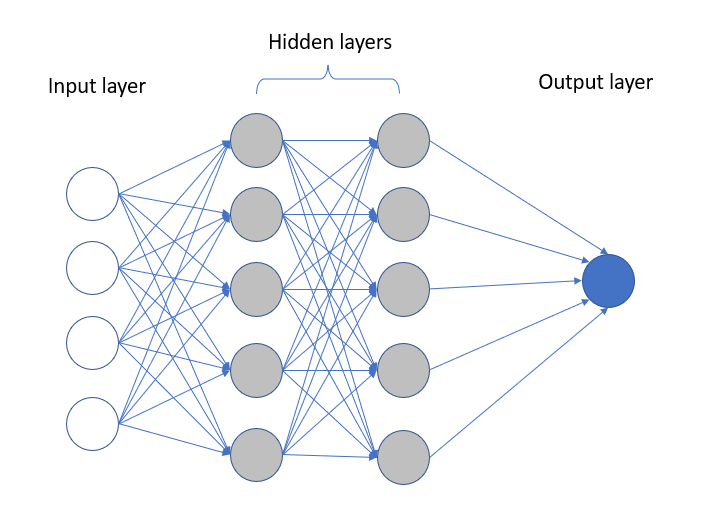Image Source: Author

## Visualizing a Neural Network using Keras Library

Now that we have discussed some basics of deep learning and neural networks, we know that deep learning models are complex, and the way they make decisions is also hard to understand. It can be interesting to visualize how a neural network connects various neurons. It can be a great way to visualize the model architecture and share it with your audience while presenting.

The Keras library allows for visualization of the neural networks using the plot_model command.

#### Creating a Neural Network Model

Before we begin this tutorial, it is expected to have a basic understanding of how to create a Neural Network. It is essential to understand the tool’s utility discussed in this article.

Let us start by creating a basic Artificial Neural Network (ANN) using Keras and its functions.

Feel free to create a different neural network since we are only visualizing the final model and hence, it might be interesting to explore the capabilities of the visual Keras library (discussed later in this article) with a different model.

```from keras.models import Sequential
from keras.layers import Dense```
```model = Sequential()
```

We can display the generated image using the following command.

```from keras.utils.vis_utils import plot_model
plot_model(model, to_file='model_plot.png', show_shapes=True, show_layer_names=True)```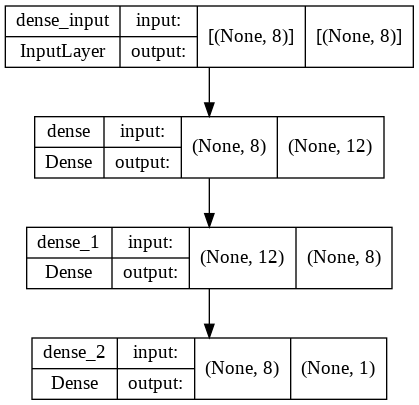From the above image, we can clearly visualize the model structure and how different layers connect with each other through a number of neurons. Next, let us build a CNN and visualize it using the Keras library.

```from keras import models
from keras.layers import Dense, Conv2D, MaxPooling2D, Flatten, Activation
from keras import layers

model = Sequential()

We can visualize this model ‘plot_model’ command used previously.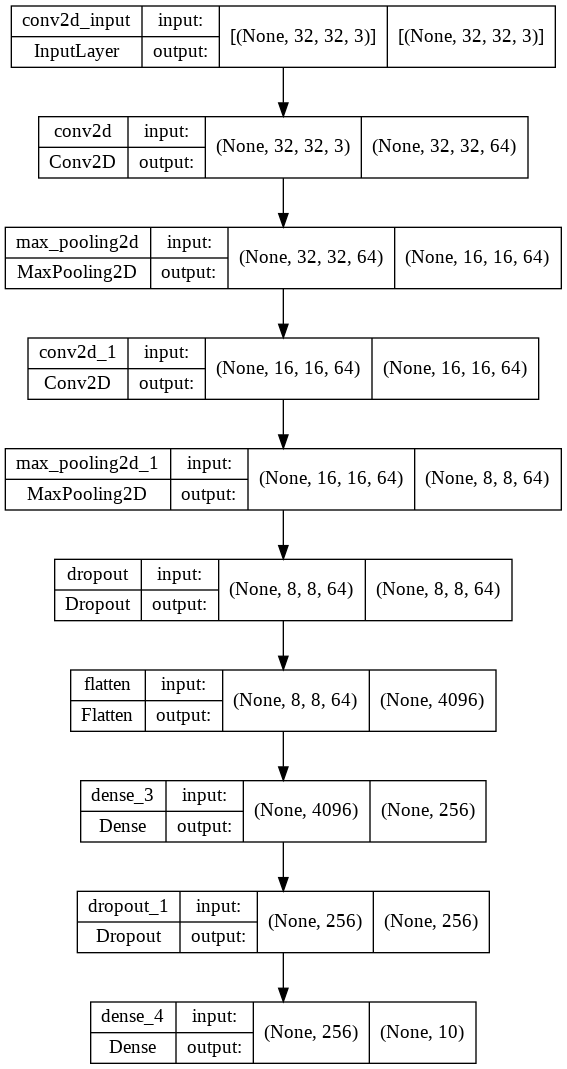Here we can visualize the different layers of the neural network along with the number of filters, filter size, no. of neurons, etc. The plot_model command from Keras library is helpful to display ANN as well as CNN structure due to its flowchart style layout of the neural network. But if there are more layers to a CNN, this visualization style requires more space and is difficult to read. There is another library, ‘Visualkeras’, which can easily help us visualize these networks. In this tutorial, we will explore the Visualkeras library and develop visualizations using it.

## Using Visualkeras to Visualize the Neural Network

Visualkeras is an open-source Python library that helps in the visualization of the Keras neural network architecture. It provides simple customization to meet a wide range of requirements. Visualkeras generates layered style architectures, which are ideal for CNNs (Convolutional Neural Networks), and graph style architectures, which are suitable for most models, including simple feed-forward networks. It’s one of the most helpful libraries for understanding how different layers are connected.

Let’s start by installing the Visualkeras library in the command prompt.

`pip install visualkeras`

Next, we will import all the libraries which are required to build a sequential model.

```import keras
import tensorflow as tf

from tensorflow import keras
from keras.models import Sequential
from tensorflow.keras.layers import Input, Conv2D, Dense, Flatten, Dropout
from tensorflow.keras.layers import GlobalMaxPooling2D, MaxPooling2D
from tensorflow.keras.models import Model
from tensorflow.keras import regularizers, optimizers```

Now we will build a simple model with some convolutional and pooling layers.

```model = Sequential()

Let’s look for the summary of the defined model.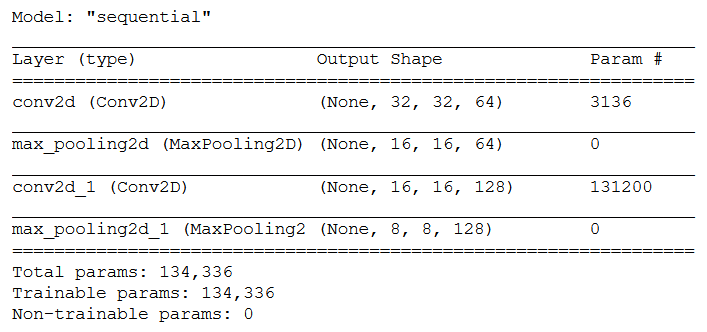Now to visualize the neural network model, we will import the Visualkeras library package as shown below.

```import visualkeras
visualkeras.layered_view(model)```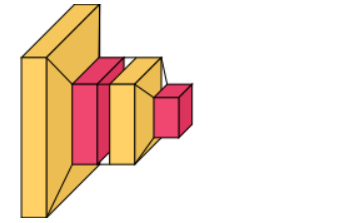The visualization of the neural network model above shows us the two different layers, i.e. convolutional layers in yellow and pooling layers in pink.

Let’s increase the complexity and add some more layers with a few dropouts to see the effect of visualization.

```model = Sequential()

The summary of the defined model is shown below.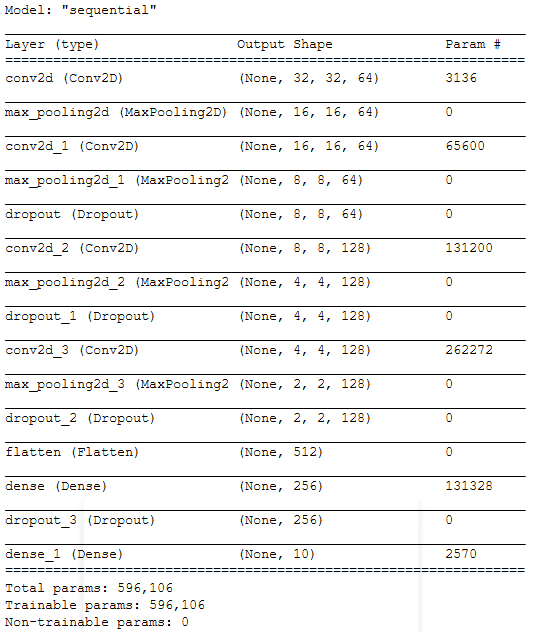Now, we visualize the model with the newly added layers.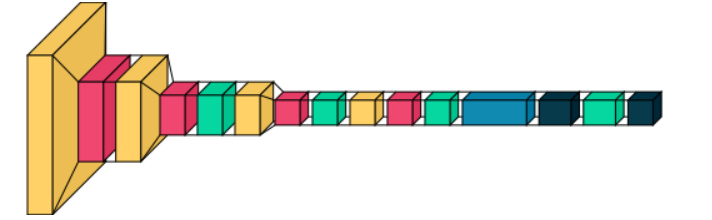Now we will add the legend to the visualization. The legend property indicates the connection between colour and layer type. It is also possible to provide a custom “PIL.ImageFont” to use otherwise, Visualkeras will use the default PIL font. Depending on your operating system, you may need to specify the entire path to the preferred font. In the case of google colab, copy the required font to the truetype font folder else, you can use the default font.

```visualkeras.layered_view(model, legend=True) # without custom font
from PIL import ImageFont
font = ImageFont.truetype("arial.ttf", 12)
visualkeras.layered_view(model, legend=True, font=font) # selected font```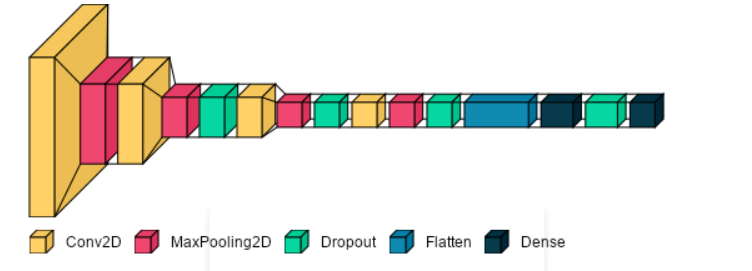Using the following code, we can see the neural network model in 2D space or in flat style.

`visualkeras.layered_view(model, legend=True, font=font, draw_volume=False)`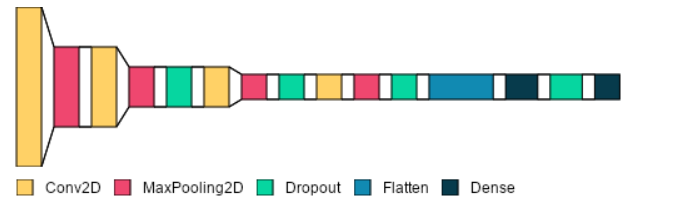The spacing between the layers can be adjusted using the ‘spacing’ variable, as shown below.

`visualkeras.layered_view(model, legend=True, font=font, draw_volume=False,spacing=30)`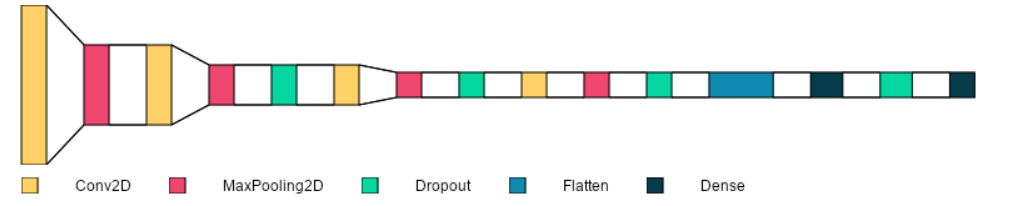We can customize the colours of the layers using the following code.

```from tensorflow.keras import layers
from collections import defaultdict
color_map = defaultdict(dict)customize the colours
color_map[layers.Conv2D]['fill'] = '#00f5d4'
color_map[layers.MaxPooling2D]['fill'] = '#8338ec'
color_map[layers.Dropout]['fill'] = '#03045e'
color_map[layers.Dense]['fill'] = '#fb5607'
color_map[layers.Flatten]['fill'] = '#ffbe0b'
visualkeras.layered_view(model, legend=True, font=font,color_map=color_map)```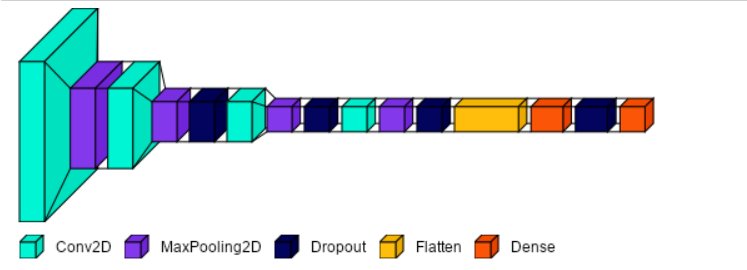Using this library, we can display any neural network layers in a convenient way with a few lines of code. This library is useful when using AutoML tools as the neural network is set up by the tool. With this library, we can easily find out details about the layers of the neural network to better understand the model through colourful visualizations.

## Summary

Visualizing neural networks is helpful to understand the connections between the layers. It is useful when we want to explain the structure of the built neural network for teaching or presenting purposes. Using a flowchart type visualization for neural networks with multiple hidden layers might be sometimes tedious to read due to space constraints. In this article, we saw an easy method to visualize a neural network using the Visualkeras library. With minimal code, we can quickly display the structure/layout of the neural network and customize it using this library. I hope you enjoyed reading this article. The code is available on my GitHub repository. Try this library for your ANN/CNN architecture and visualize the neural network better.

#### Author Bio

Devashree holds an M.Eng degree in Information Technology from Germany and a background in Data Science. She likes working with statistics and discovering hidden insights in varied datasets to create stunning dashboards. She enjoys sharing her knowledge in AI by writing technical articles on various technological platforms.
She loves travelling, reading fiction, solving Sudoku puzzles, and participating in coding competitions in her leisure time.•